Questions - Forming equations and solving

Chapter 12 Class 12 Linear Programming
Serial order wise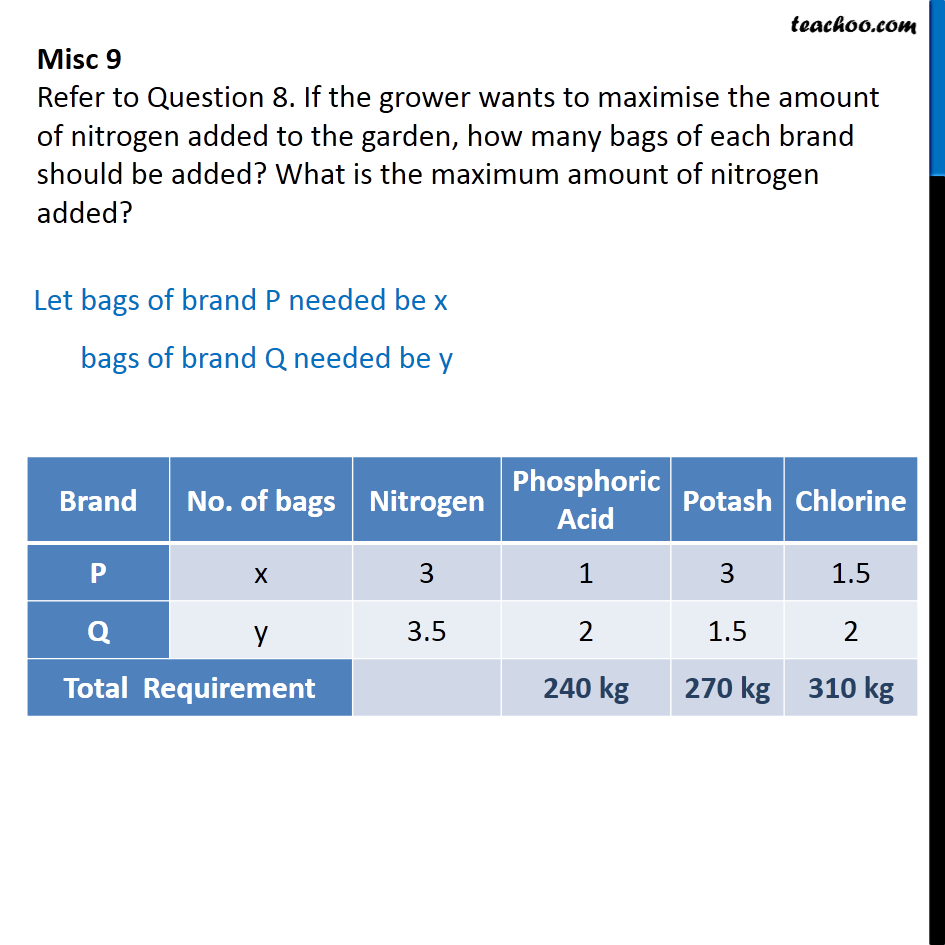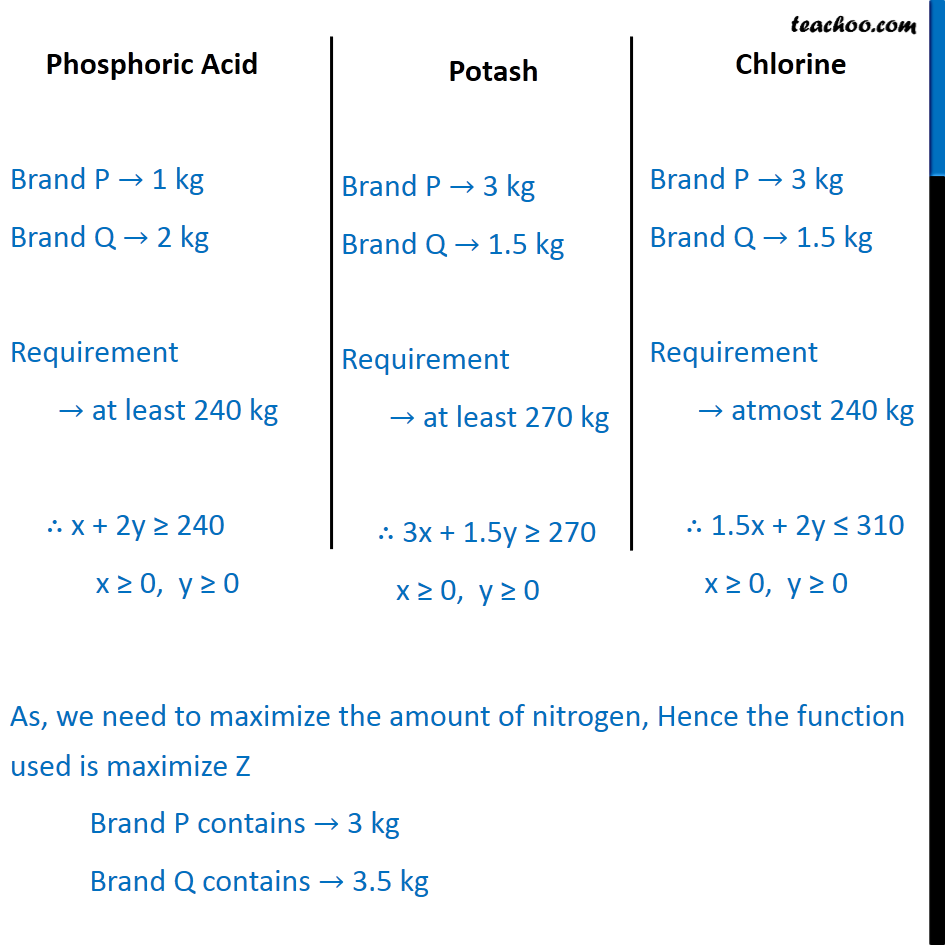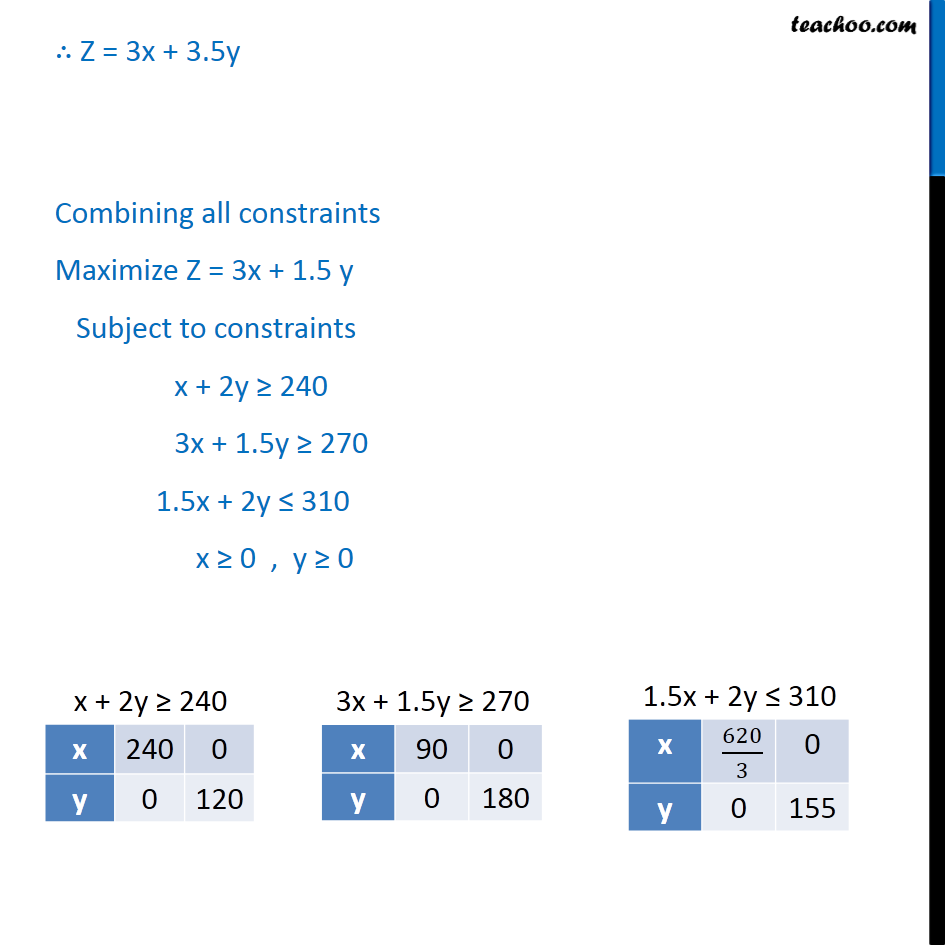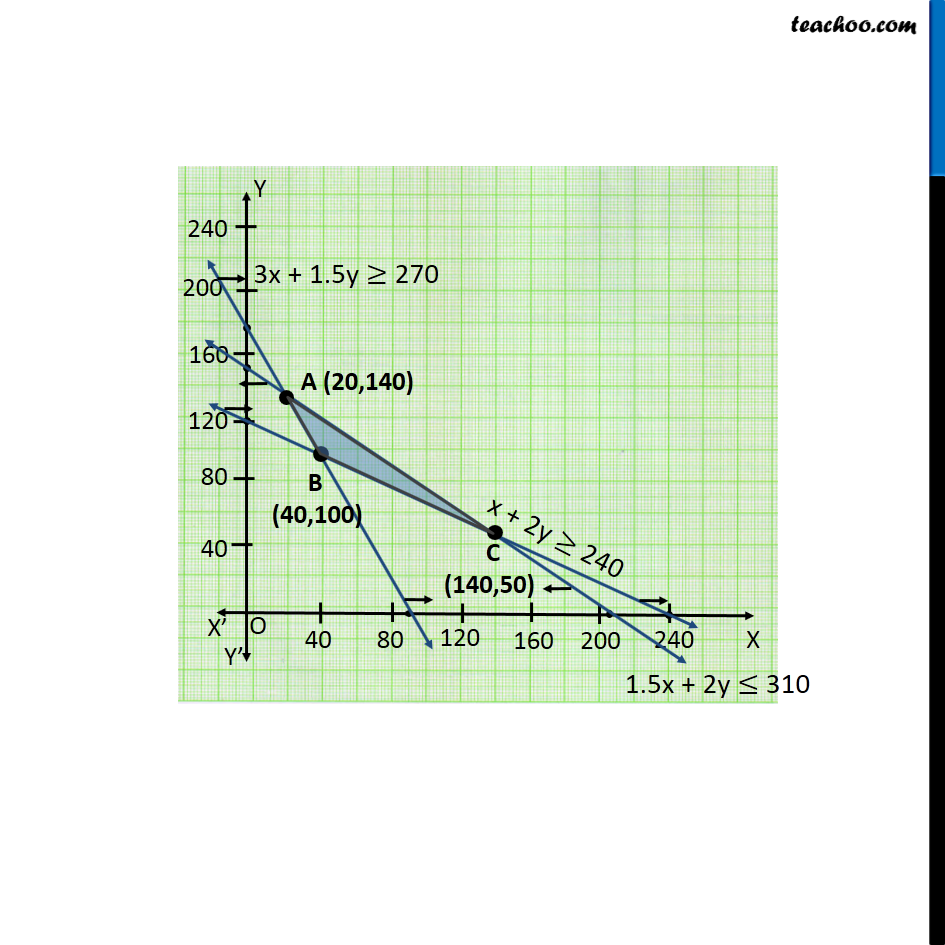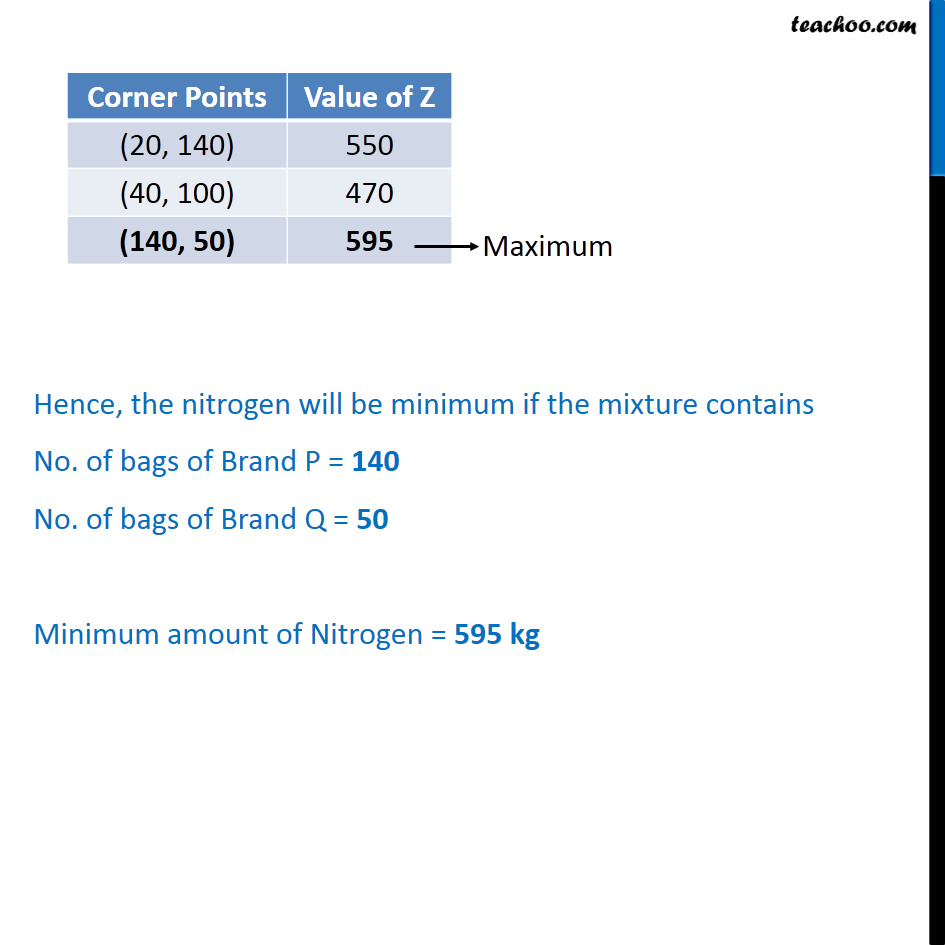Learn in your speed, with individual attention - Teachoo Maths 1-on-1 Class

### Transcript

Misc 9 Refer to Question 8. If the grower wants to maximise the amount of nitrogen added to the garden, how many bags of each brand should be added? What is the maximum amount of nitrogen added? Let bags of brand P needed be x bags of brand Q needed be y As, we need to maximize the amount of nitrogen, Hence the function used is maximize Z Brand P contains → 3 kg Brand Q contains → 3.5 kg ∴ Z = 3x + 3.5y Combining all constraints Maximize Z = 3x + 1.5 y Subject to constraints x + 2y ≥ 240 3x + 1.5y ≥ 270 1.5x + 2y ≤ 310 x ≥ 0 , y ≥ 0 Hence, the nitrogen will be minimum if the mixture contains No. of bags of Brand P = 140 No. of bags of Brand Q = 50 Minimum amount of Nitrogen = 595 kg